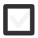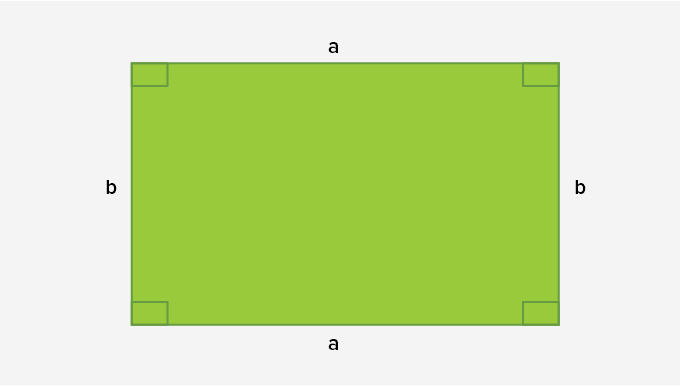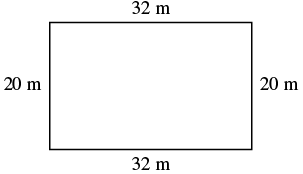# Can calculate a rectangles perimeter and area that is 2m high and 3m wide.+1 vote
265 views

## 1 lesson

+1 vote

A perimeter of a geometric shape would be the path we would have to travel if we would walk around the shape on its edges.
Simply speaking in order to figure out a perimeter of a geometric shape we need to know the lenght of all of its edges and sum these lengths together.

Irregular geometric shapes make it difficult as we have to know each and every edge in order to make the calculation. So if have a irregular geometric shape with four diffrent egdes : a,b,c,d... The perimetire would simply be:

P = a + b + c + d

A good example of this you can find on this link

With regular geometric shapes it is a little bit easier as they follow some rules. For example if we take rectangle, it is a geometric shape that is by definition made of four egdes, out of two and two are equal.Image sourced: https://www.splashlearn.com/math-vocabulary/geometry/rectangle

Taking that into account, we would write that perimeter of a rectangle is a sum of its four egdes:

P = a + a + b + b

or simpified

P = 2 x a + 2 x b

Which makes it a lot easier.

Let's try it on an examle.
Let's try and find a perimeter of some rectangles.Here we can se we have edge a= 32 m and edge b= 20 m (here we also use units which doesn't change things a lot).

So our perimeter should be the sum of all edges.

P = 2 x a + 2 x b
P = 2 x 32m + 2 x 20m = 64m + 40m
P = 104m

So our perimeter is 104 m and this is the procudere we will use whenever we have to calculate perimiter of a rectangle.

To find out more about calculating perimeter check out this interesting video: